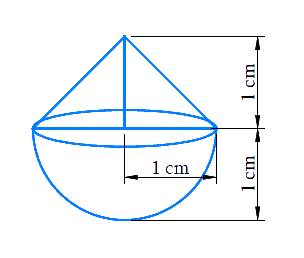# Ex.13.2 Q1 Surface Areas and Volumes Solution - NCERT Maths Class 10

Go back to  'Ex.13.2'

## Question

A solid is in the shape of a cone standing on a hemisphere with both their radii Being equal to $$1\,\rm{cm}$$ and the height of the cone is equal to its radius. Find the volume of the solid in terms of $$\pi$$.

Video Solution
Surface Areas And Volumes
Ex 13.2 | Question 1

## Text Solution

What is known?

A solid in the shape of a cone standing on a hemisphere. Radius and height of the cone are same as the radius of the hemisphere which is $$1\rm{cm}$$.

What is unknown?

The volume of the solid.

Reasoning:

Draw a figure of the solid to visualize itSince the solid is made up of conical part and a hemispherical part.

Volume of the solid $$=$$ volume of the conical part $$+$$ volume of the hemispherical part

We will find the volume of the solid by using formulae;
Volume of the hemisphere  \begin{align} = \frac{2}{3}\pi {r^3}\end{align}
where $$r$$ is the radius of the hemisphere
Volume of the cone   \begin{align} = \frac{1}{3}\pi {r^2}h\end{align}
where $$r$$ and $$h$$ are the radius and height of the cone respectively.

Steps:

Radius of hemispherical part $$=$$ Radius of conical part $$=r=1\,\rm{cm}$$

Height of conical part $$= h = r = 1 \rm cm$$

Volume of the solid  $$=$$ volume of the conical part $$+$$ volume of the hemispherical part

\begin{align}&= \frac{1}{3}\pi {r^2}h + \frac{2}{3}\pi {r^3}\\&= \frac{1}{3}\pi {r^3} + \frac{2}{3}\pi {r^3}\\&= \pi {r^3}\\ &= \pi {\left( {1 \rm cm} \right)^3}\\&= \pi \rm c{m^3}\end{align}

Learn from the best math teachers and top your exams

• Live one on one classroom and doubt clearing
• Practice worksheets in and after class for conceptual clarity
• Personalized curriculum to keep up with school